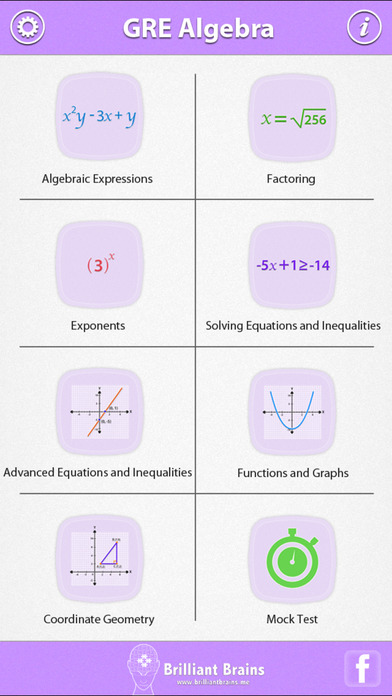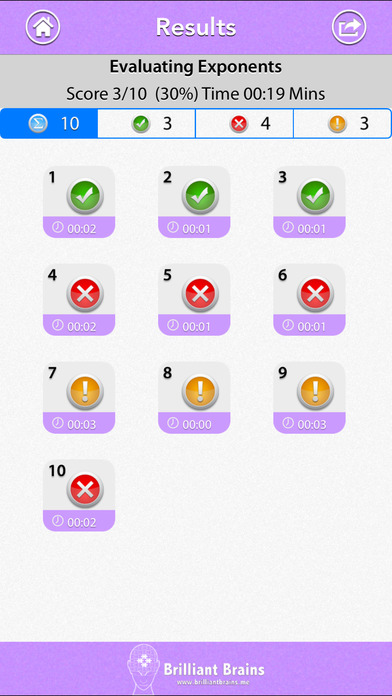GRE Math : Algebra Review

iOS UniversalEducation

\$1.99

Prepare to achieve the GRE scores you need to get into your top choice schools.
Get ready for the test day with this most comprehensive app on GRE Math : Algebra Review.

Features include:

** HIGHEST QUALITY and QUANTITY
Over 440 questions and 44 revision notes in all just for Algebra Review. High quality content written by experienced GRE tutors.

** MOCK TEST
Mixed questions from all topics.

** INSTANT FEEDBACK
Know instantly if your answer was right or wrong.

** REVIEW WITH EXPLANATION
Review each question at the end of the test. Know the right answer with detailed explanation for each question.

** PROGRESS METER
With our unique progress tracking feature including pie charts and bar graphs showing your progress, you know you are ready to take on the real test at the board when your progress meter says 100%.

** REVISE BY TOPIC
1. Algebraic Expression
Translation into Algebraic Expressions
Addition and Subtraction of Algebraic Expressions
Multiplication of Algebraic Expressions
Expanding Simplifying and Factoring
Evaluating Expressions 1
Evaluating Expressions 2
Binomial Expansion
Algebraic Fractions
Manipulation and Evaluation of Expressions

2. Factoring
Finding Common Factors
Factoring Quadratics with Unit Coefficient
Difference of Two Squares

3. Exponents
Evaluating Exponents
Multiplying Exponents
Dividing Exponents
Exponent of a Exponent

4. Solving Equations & Inequalities
Linear Equations 1
Linear Equations 2
Linear Equations 3
Setting up Equations
Inequalities
Solving Inequalities

5. Advanced Equations & Inequalities
Systems of Equations (Linear)
Setting up Systems of Equations
Using the Quadratic Formula
Solving Quadratics by Factoring
Rational Equations and Inequalities

6. Functions and Graphs
Linear Graphs
Slope
Find the Equation of a Line from its Graph
Using Graphs to Solve Linear Simultaneous Equations
Graphical Inequalities
Domain Range Evaluation
Functions as Models
Translations of a Graph
Circles and Parabolas

7. Coordinate Geometry
Geometry in the Coordinate Plane
Line Lengths and Midpoints
Parallel and Perpendicular Lines
Points in the Coordinate Plane

Disclaimer:
GRE is a registered trademark of Educational Testing Services(ETS). ETS neither sponsors nor endorses this product or any of its content.

What's New

Updated data and code to work with latest iOS version.

•••••